# (4) Consider the 2 x 2 matrices 1-69). -:- (-1). «-6 -1) :-(1) (a) Prove that...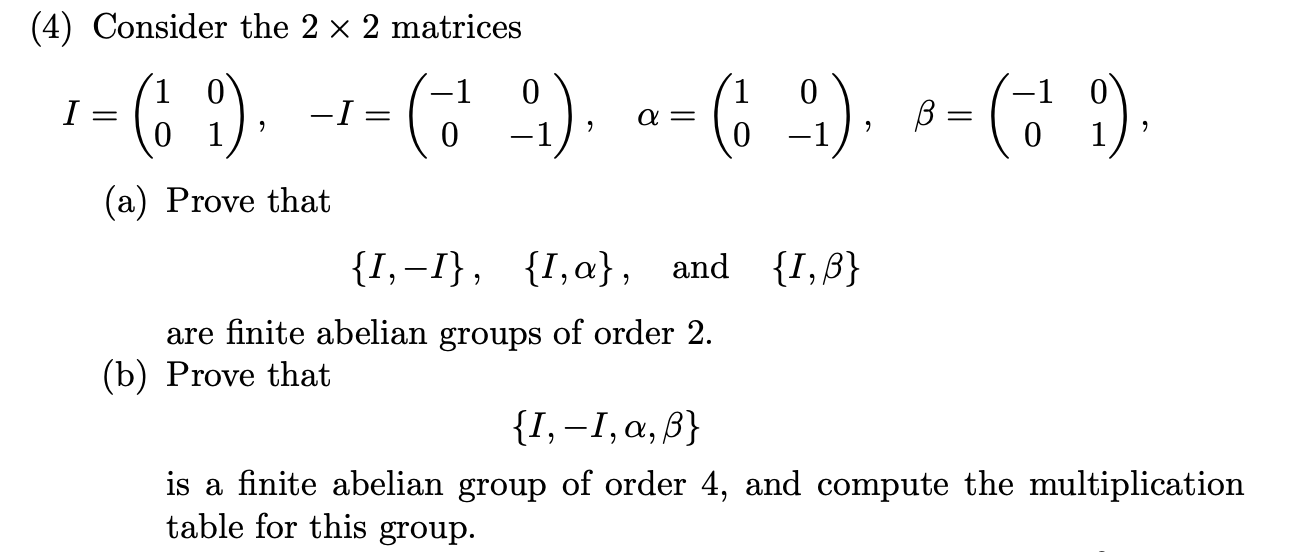(4) Consider the 2 x 2 matrices 1-69). -:- (-1). «-6 -1) :-(1) (a) Prove that {1,-1}, {1,a}, and {1,3} are finite abelian groups of order 2. (b) Prove that {1,-1,a,ß} is a finite abelian group of order 4, and compute the multiplication table for this group.

Solution: Given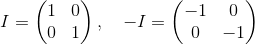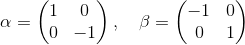Now,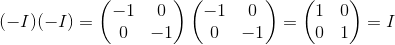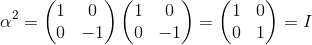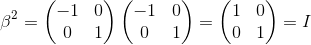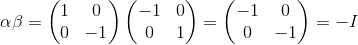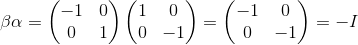a).

Now , the composition tables for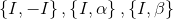are respectively below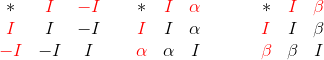From above composition tables it is clear thatare closed.are associative.all have identity element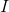.

and inverse of each element is itself.

also all elements in composition tables commute to each other.

Thus,are abelian groups with 2 elements.

Hence,are finite abelian groups of order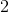.

b).

Now, the composition table for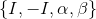is below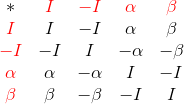From above composition tables it is clear thatis closed.is associative.has identity element.

and inverse of each element is itself.

also all elements in composition tables commute to each other.

Thus,are abelian groups with 4 elements.

Hence,are finite abelian groups of order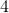.

Which is the required proof.

This complete the solution.

##### Add Answer of: (4) Consider the 2 x 2 matrices 1-69). -:- (-1). «-6 -1) :-(1) (a) Prove that...
Similar Homework Help Questions
• ### 4. Let M be the set of 2 x 2 matrices of the form (62) where...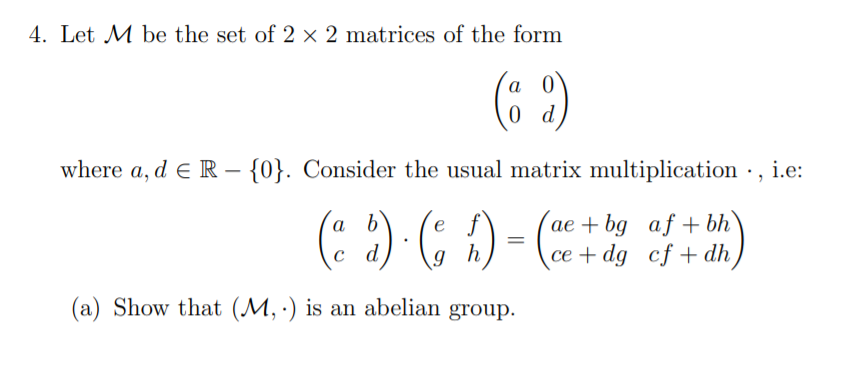4. Let M be the set of 2 x 2 matrices of the form (62) where a, d E R - {0}. Consider the usual matrix multiplication ·, i.e: ae + bg af + bh ce + dg cf + dh (a) Show that (M,·) is an abelian group. 1 (b) Compute the cyclic subgroup generated by M = What is the order of M? 66 -4) (1) EM EM.

• ### Let M be the set of 2 x 2 matrices of the form (82) where a,...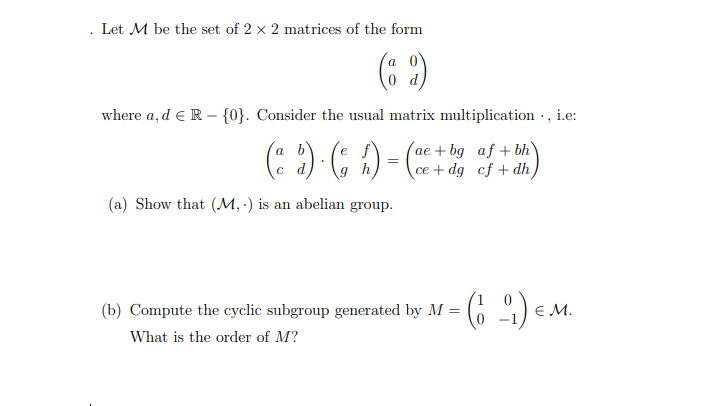Let M be the set of 2 x 2 matrices of the form (82) where a, d ER-{0}. Consider the usual matrix multiplication, i.e: ae + bg af +bh ce + dg cf + dh (2)) = (ce ) (a) Show that (M,-) is an abelian group. (b) Compute the cyclic subgroup generated by M = What is the order of M? (6 -4) € M.

• ### Problem 3. Consider the general linear group GL2 = (M2,*) of 2 x 2 invertible matrices...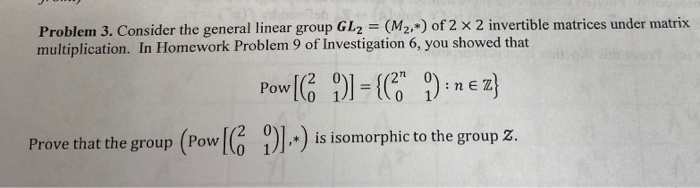Problem 3. Consider the general linear group GL2 = (M2,*) of 2 x 2 invertible matrices under matrix multiplication. In Homework Problem 9 of Investigation 6, you showed that Pow G 1-( )z is isomorphic to the group Z. Prove that the group (Pow 1 i

• ### 1. Prove that the matrices are hermitian or anti-hermitian. 2. Prove that in order for the...

1. Prove that the matrices are hermitian or anti-hermitian. 2. Prove that in order for the Dirac equation to be covariant (i.e., form-invariant) under the Lorentz group of trans formations with the constraint that the gamma-matrices are unchanged, the following relation must hold: , where is the Lorentz-transformation matrix for the 4- vectors (x'=Lx) and S is a unitary matrix that transforms the spinor as

• ### #21. Let G be the set of all real 2 x 2 matrices where ad +...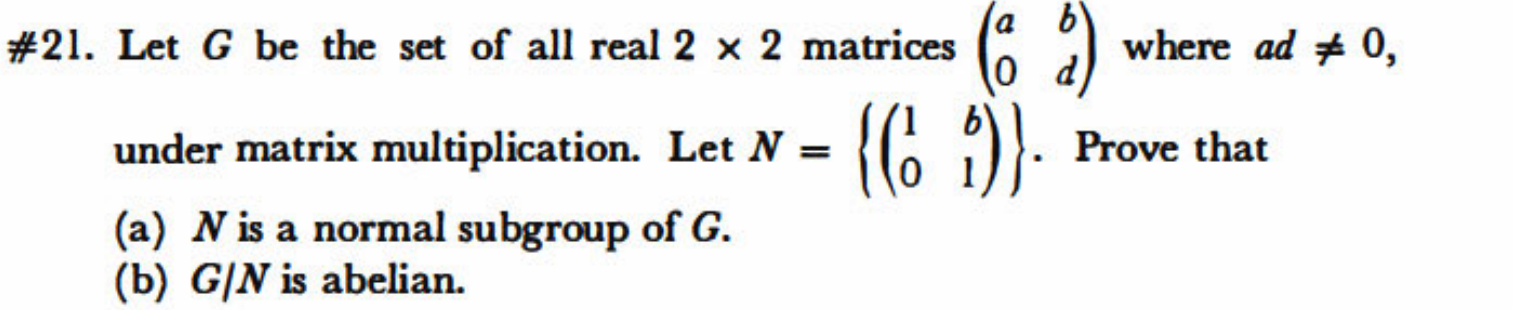#21. Let G be the set of all real 2 x 2 matrices where ad + 0, Prove that under matrix multiplication. Let N = (a) N is a normal subgroup of G. (b) G/N is abelian.

• ### 2. Consider the set S-[1, oo). Consider the open cover x(n-1,n+)InEN) - (0,2),(1,3),(2.4),(8,5.,..) of S. Prove that X contains no finite subcover of S. Hence S is not compact. 2. Consider t...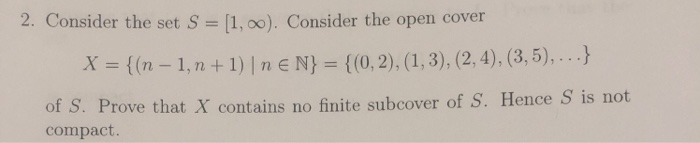2. Consider the set S-[1, oo). Consider the open cover x(n-1,n+)InEN) - (0,2),(1,3),(2.4),(8,5.,..) of S. Prove that X contains no finite subcover of S. Hence S is not compact. 2. Consider the set S-[1, oo). Consider the open cover x(n-1,n+)InEN) - (0,2),(1,3),(2.4),(8,5.,..) of S. Prove that X contains no finite subcover of S. Hence S is not compact.

• ### 1- (2,5+2,5 mark) Consider in GL(2, Q), the subset (a=1 or a=-1),bez Prove that H, with multiplication, is a subgroup of GL(2,Q) a) Is the function b) an homomorphism of groups? Justify your answ...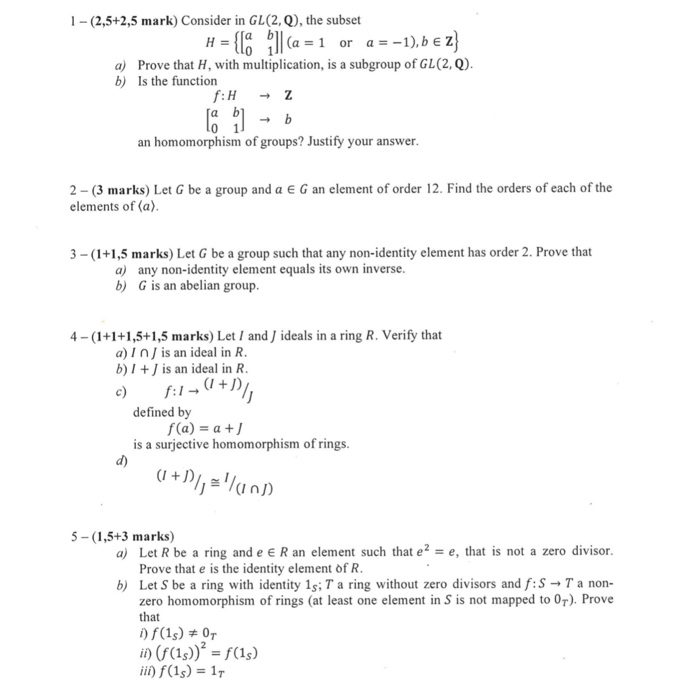1- (2,5+2,5 mark) Consider in GL(2, Q), the subset (a=1 or a=-1),bez Prove that H, with multiplication, is a subgroup of GL(2,Q) a) Is the function b) an homomorphism of groups? Justify your answer 2 (3 marks) Let G be a group and a E G an element of order 12. Find the orders of each of the elements of (a) 3- (1+1,5 marks) Let G be a group such that any non-identity element has order 2. Prove that a)...

• ### 2. Consider the following set of complex 2 x 2 matrices where i = -1: H...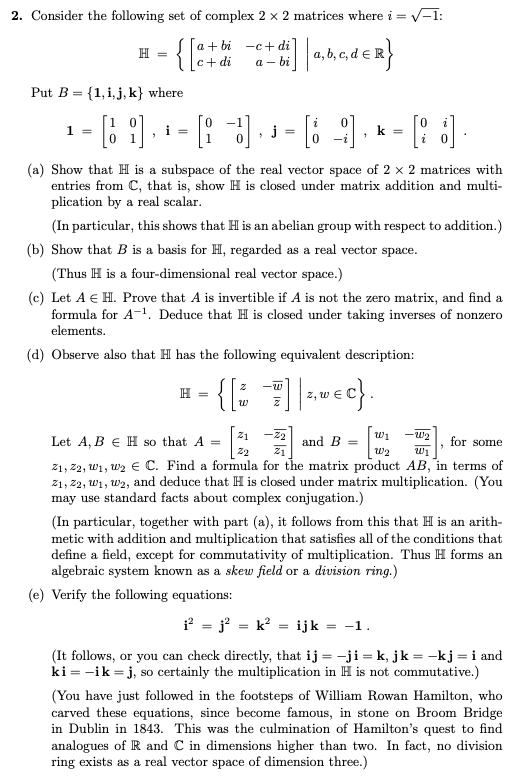2. Consider the following set of complex 2 x 2 matrices where i = -1: H = a + bi -c+dil Ic+dia-bi Put B = {1, i, j, k} where = = {[ctdie met di]|1,3,c,dex} 1-[ ), : = [=]. ; = [i -:], « =(: :] . (a) Show that H is a subspace of the real vector space of 2 x 2 matrices with entries from C, that is, show H is closed under matrix addition and multi-...

• ### 5. Prove that the center of a group is the intersection of all the centralizers. 6....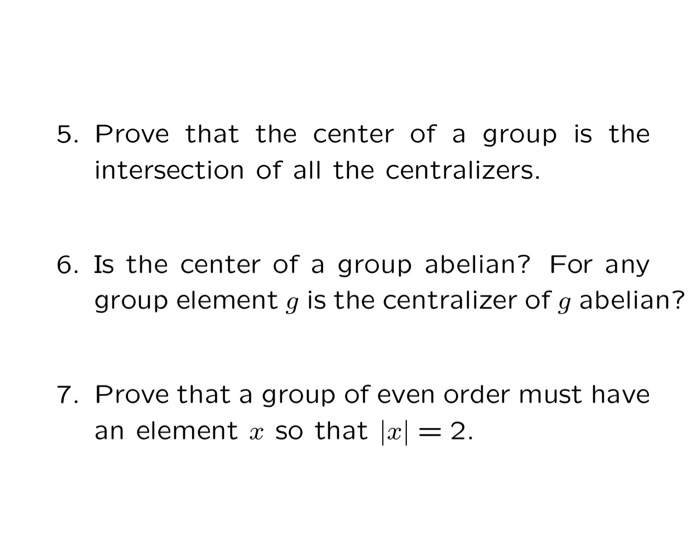5. Prove that the center of a group is the intersection of all the centralizers. 6. Is the center of a group abelian? For any group element g is the centralizer of g abelian? 7. Prove that a group of even order must have an element x so that x= 2.

• ### (more questions will be posted today in about 6 hrs from now.) December 8, 2018 WORK ALL PROBLEMS. SHOW WORK & INDICATE REASONING \ 1.) Let σ-(13524)(2376)(4162)(3745). Express σ as a product...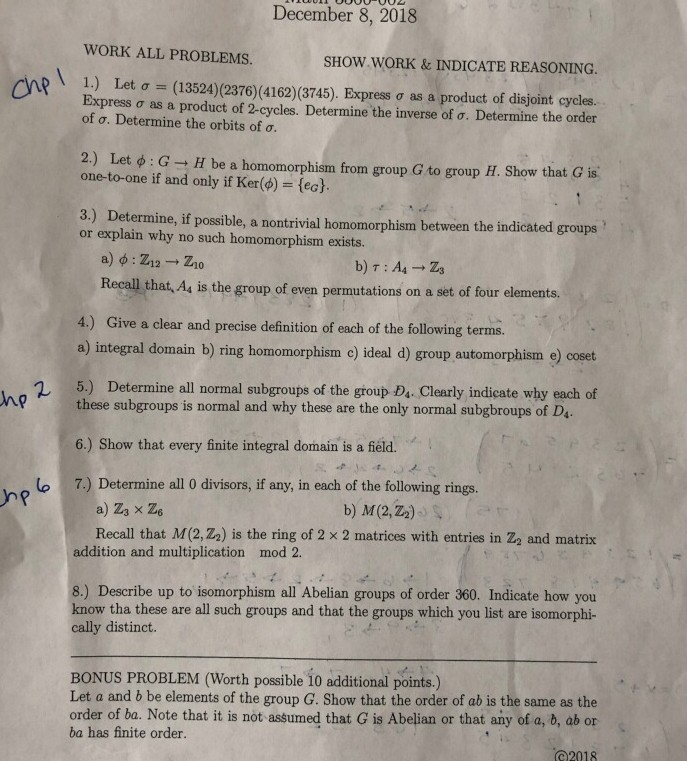(more questions will be posted today in about 6 hrs from now.) December 8, 2018 WORK ALL PROBLEMS. SHOW WORK & INDICATE REASONING \ 1.) Let σ-(13524)(2376)(4162)(3745). Express σ as a product of disjoint cycles Express σ as a product of 2 cycles. Determine the inverse of σ. Determine the order of ơ. Determine the orbits of ơ 2) Let ф : G H be a homomorphism from group G to group H. Show that G is. one-to-one if and...

Free Homework App